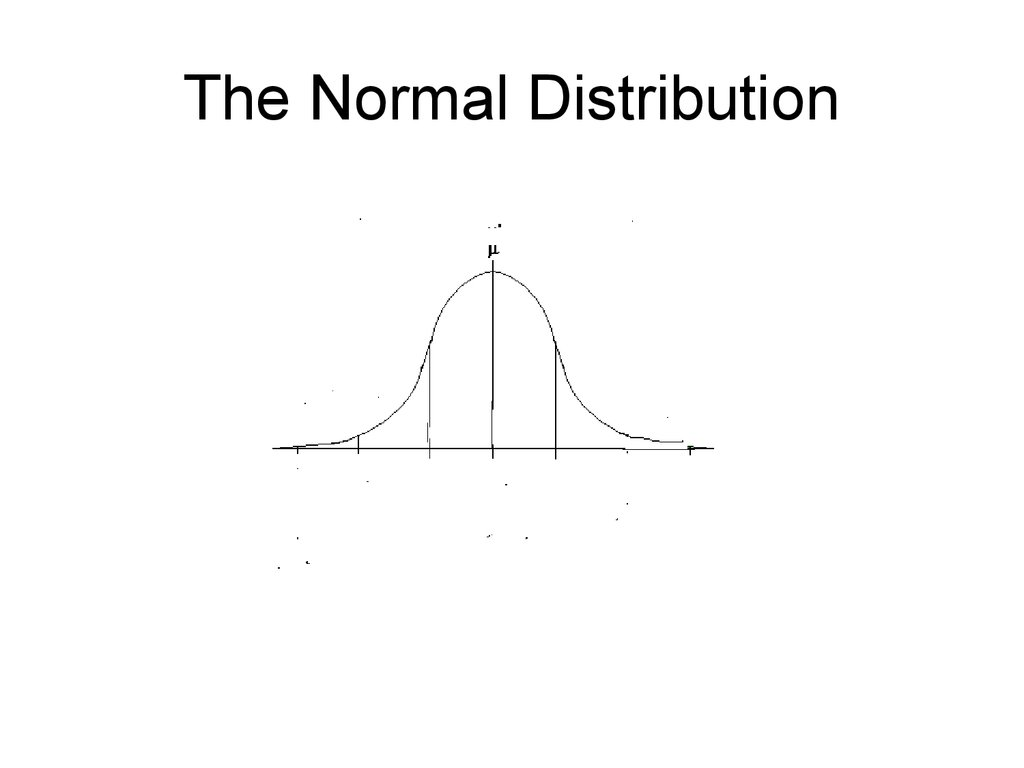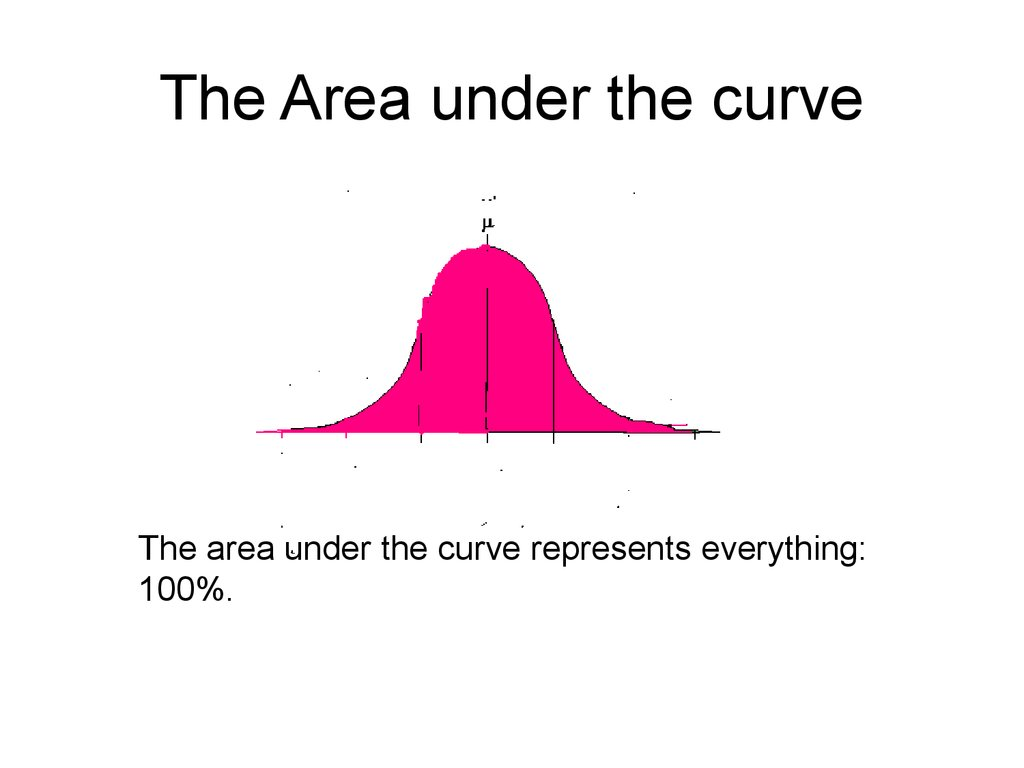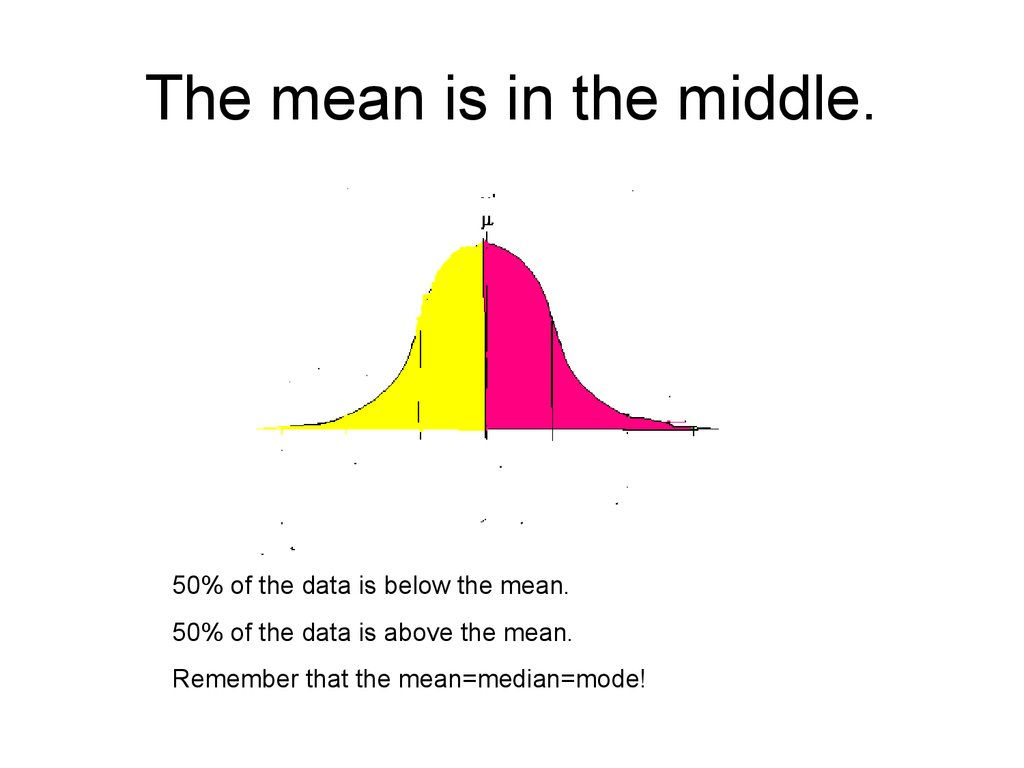# The normal distribution

## 2. The Area under the curve

The area under the curve represents everything:
100%.

## 3. The mean is in the middle.

50% of the data is below the mean.
50% of the data is above the mean.
Remember that the mean=median=mode!

P(-1<Z<1) = 68%

68%
P(0<Z<1)
P(z<1)
P(z>1)

## 6. Part (Yellow) + Part (Brown) =100

100 - Part (Yellow) = Part (Brown)
100 – 84 = 16

P(-2<Z<2)
P(1 < Z < 2)
P(Z < 2)

## 8. The Normal Distribution

A normal curve is bell shaped.
The highest point on the curve
is the mean of the distribution.
The mean, median and mode
are the same.
The curve is symmetric with
respect to its mean.
The total area under the curve
is one.
Roughly 68% of the data is
within one standard deviation
from the mean, 95% of the
data are within two standard
deviations and 99.7% are
within three standard
deviations.

## 9. Example 1

1,000 students take an
intelligence test
• The mean is 450 and the
standard deviation is 25.
• Label the horizontal axis.
• Show the Rule for the intervals
for within 1 standard deviation,
within 2 and within 3.
What percent of the data would
be between 425 and 475?
How many scores would be
between 425 and 475?
375 400 425 450 475 500 525

## 10. Label the bell!

The mean value of land and buildings
per acre from a sample of farms is
\$1000 with a standard deviation of
\$200. The data distribution has a bell
shape. Estimate the percent of farms
whose land and building values per acre
are between \$800 and \$1200.

## 11. Label the bell!

• The mean value of land and buildings per
acre from a sample of farms is \$1200 with
a standard deviation of \$350. Between
what two values does about 95% of the
data lie?

## 12. Label the bell!

• The mean price of new homes from a
sample of houses is \$155,000 with a
standard deviation of \$15,000. The data
has a bell shaped distribution.
• Between what two prices do 95% of the
houses fall?
• What is the median price?
• What percent is less than \$110,000?

## 13. Convert x to z

• Z is the standardized
value
(x )
• Z=
• Convert x = 55 with a
mean of 50 and the
standard deviation of
10.

## 14. The Calculator Finding P(a<x<b)

The Calculator
Finding P(a<x<b)
• 2nd VARS DISTR Normalcdf
In words:
(lower limit, upper limit, mean, standard deviation)
In variables:
(a, b, µ, σ)
For example with an x:
Find the probability that x is between 40 and 60 in
a distribution with a mean of 50 and a standard
deviation of 10.
P(40<x<60) = normalcdf(40, 60, 50,10)

## 15. Write the normalcdf for each µ=50 and σ=10

• P(20<x<60)= normalcdf(___,___,___,___)
• P(20<x<50)= normalcdf(___,___,___,___)
• P(70<x<80)= normalcdf(___,___,___,___)
• P(14<x<43)= normalcdf(___,___,___,___)

## 16. ∞ = 1E99 -∞ = -1E99

∞ = 1E99
-1E99
-∞ =
• P(50<x<∞)= normalcdf(___,___,___,___)
• P(55<x< ∞)= normalcdf(___,___,___,___)
• P(-∞ <x<30)= normalcdf(___,___,___,___)
• P(-∞ < x< 60)= normalcdf(___,___,___,___)

## 17. Let’s Do A Graph

• Normal curves are graphed by normalpdf